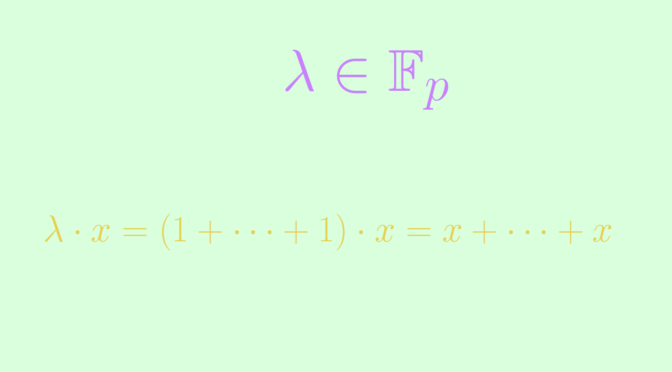# Additive subgroups of vector spaces

Consider a vector space $$V$$ over a field $$F$$. A subspace $$W \subseteq V$$ is an additive subgroup of $$(V,+)$$. The converse might not be true.

If the characteristic of the field is zero, then a subgroup $$W$$ of $$V$$ might not be an additive subgroup. For example $$\mathbb R$$ is a vector space over $$\mathbb R$$ itself. $$\mathbb Q$$ is an additive subgroup of $$\mathbb R$$. However $$\sqrt{2}= \sqrt{2}.1 \notin \mathbb Q$$ proving that $$\mathbb Q$$ is not a subspace of $$\mathbb R$$.

Another example is $$\mathbb Q$$ which is a vector space over itself. $$\mathbb Z$$ is an additive subgroup of $$\mathbb Q$$, which is not a subspace as $$\frac{1}{2} \notin \mathbb Z$$.

Yet, an additive subgroup of a vector space over a prime field $$\mathbb F_p$$ with $$p$$ prime is a subspace. To prove it, consider an additive subgroup $$W$$ of $$(V,+)$$ and $$x \in W$$. For $$\lambda \in F$$, we can write $$\lambda = \underbrace{1 + \dots + 1}_{\lambda \text{ times}}$$. Consequently $\lambda \cdot x = (1 + \dots + 1) \cdot x= \underbrace{x + \dots + x}_{\lambda \text{ times}} \in W.$

Finally an additive subgroup of a vector space over any finite field is not always a subspace. For a counterexample, take the non-prime finite field $$\mathbb F_{p^2}$$ (also named $$\text{GF}(p^2)$$). $$\mathbb F_{p^2}$$ is also a vector space over itself. The prime finite field $$\mathbb F_p \subset \mathbb F_{p^2}$$ is an additive subgroup that is not a subspace of $$\mathbb F_{p^2}$$.